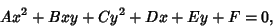(1)

the quantityis known as the discriminant, where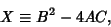(2)

and is invariant under Rotation. Using the Coefficients from Quadratic Equations for a rotation by an angle,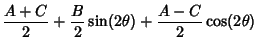(3)(4)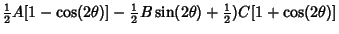(5)

Now let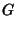(6)(7)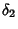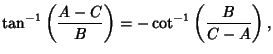(8)

and use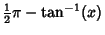(9)(10)

to rewrite the primed variables(11)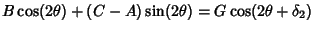(12)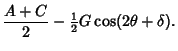(13)

From (11) and (13), it follows that(14)

Combining with (12) yields, for an arbitrary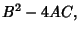(15)

which is therefore invariant under rotation. This invariant therefore provides a useful shortcut to determining the shape represented by a Quadratic Curve. Choosingto make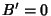(see Quadratic Equation), the curve takes on the form(16)

Completing the Square and defining new variables gives(17)

Without loss of generality, take the sign ofto be positive. The discriminant is(18)

Now, if, thenand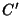both have the same sign, and the equation has the general form of an Ellipse (ifandare positive). If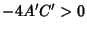, thenandhave opposite signs, and the equation has the general form of a Hyperbola. If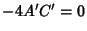, then eitheroris zero, and the equation has the general form of a Parabola (if the Nonzerooris positive). Since the discriminant is invariant, these conclusions will also hold for an arbitrary choice of, so they also hold whenis replaced by the original. The general result is
1. If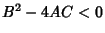, the equation represents an Ellipse, a Circle (degenerate Ellipse), a Point (degenerate Circle), or has no graph.

2. If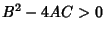, the equation represents a Hyperbola or pair of intersecting lines (degenerate Hyperbola).

3. If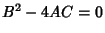, the equation represents a Parabola, a Line (degenerate Parabola), a pair of Parallel lines (degenerate Parabola), or has no graph.